# Talented Physics Teacher Mind-Blowing Diagrams Makes Art Out of Formulas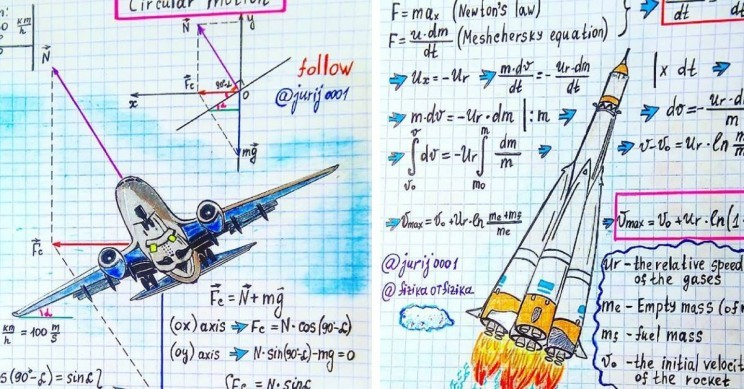Yuri Kovalenok is a talented physics teacher that makes art out of formulas. From Circular motion to the law of Electromagnetism, this teacher-turned-artist illustrates all your favourite formulas.

For today we picked top Five of our favourites, Yuri Kovalenok Art, to share with you. You can find plenty more on his Instagram account.

### 1. Circular motion

Kovalenok calls this one “Centripetal acceleration and force” and describes how it refers to an airplane making a turn. “The aircraft makes a turn, moving in an arc of a circle with a constant speed v=360 km/h. Determine the radius R of the circle, if the body of the aircraft is rotated around the direction of flight at an angle of 10 degrees,” says his Instagram post’s description.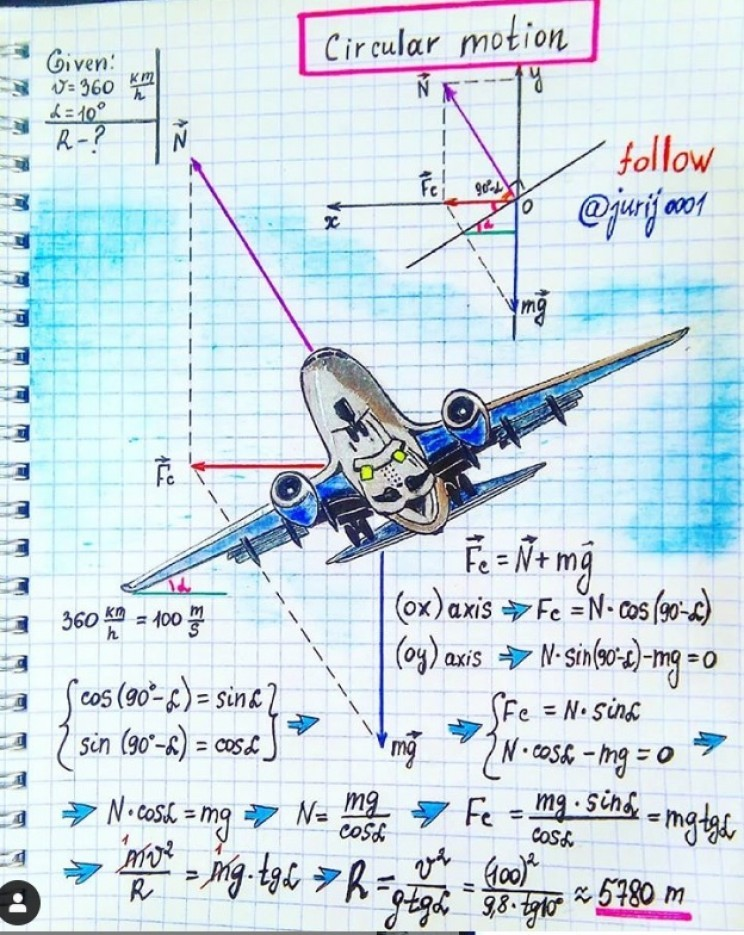### 2. Artificial satellite rotation

Have you ever wondered about the motion of artificial satellites? Well, Kovalenok is here to explain it and illustrate it. He also offers a little pop quiz. “The period of rotation of the satellite around the Earth is 24 hours. Find at what altitude is the orbit of the satellite?” says this post’s description. Can you find the altitude?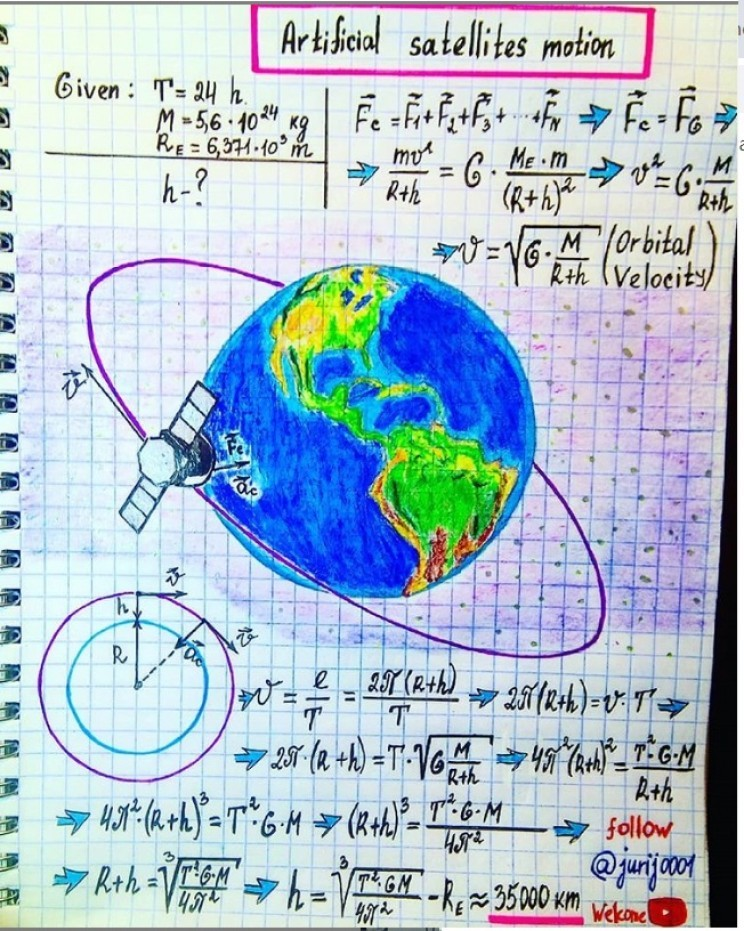### 3. Tsiolkovsky rocket equation

Tsiolkovsky’s rocket equation is a mathematical equation that describes the motion of vehicles that follow the basic principle of a rocket. It is illustrated here with an actual rocket along with the equations.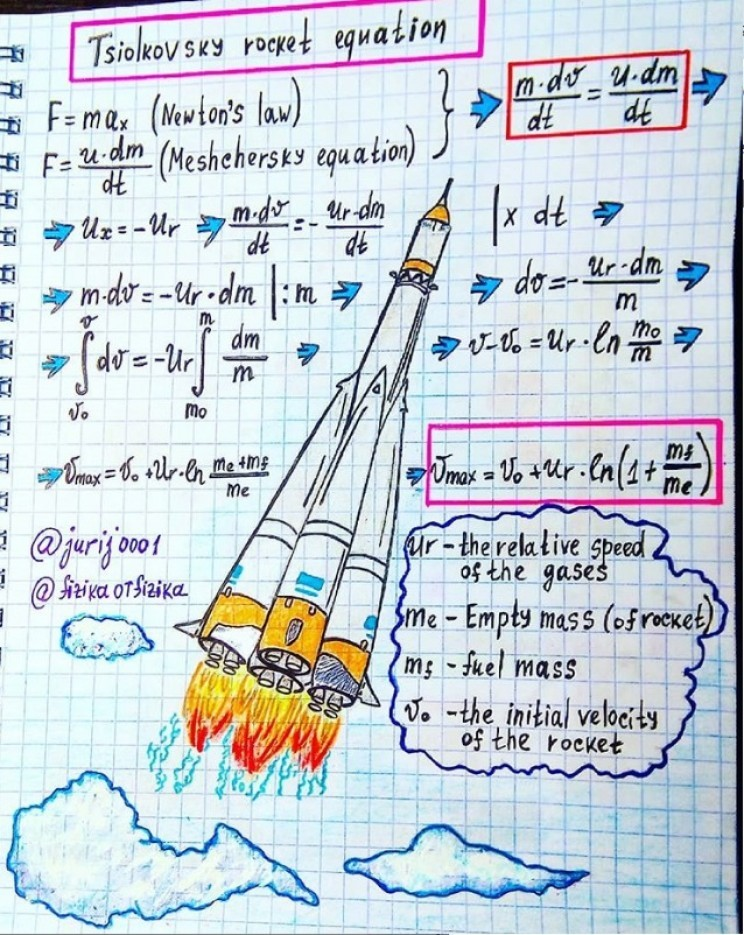### 3. Law of conservation of energy

What a perfect way to illustrate such an abstract concept as the law of conservation of energy. Kovalenok even gives a description for his drawing: “In the experiment with the “dead loop” the ball of mass m is released from height h=3R ( where R is the radius of the loop ). With what force presses the ball in the lower and upper points of the loop? “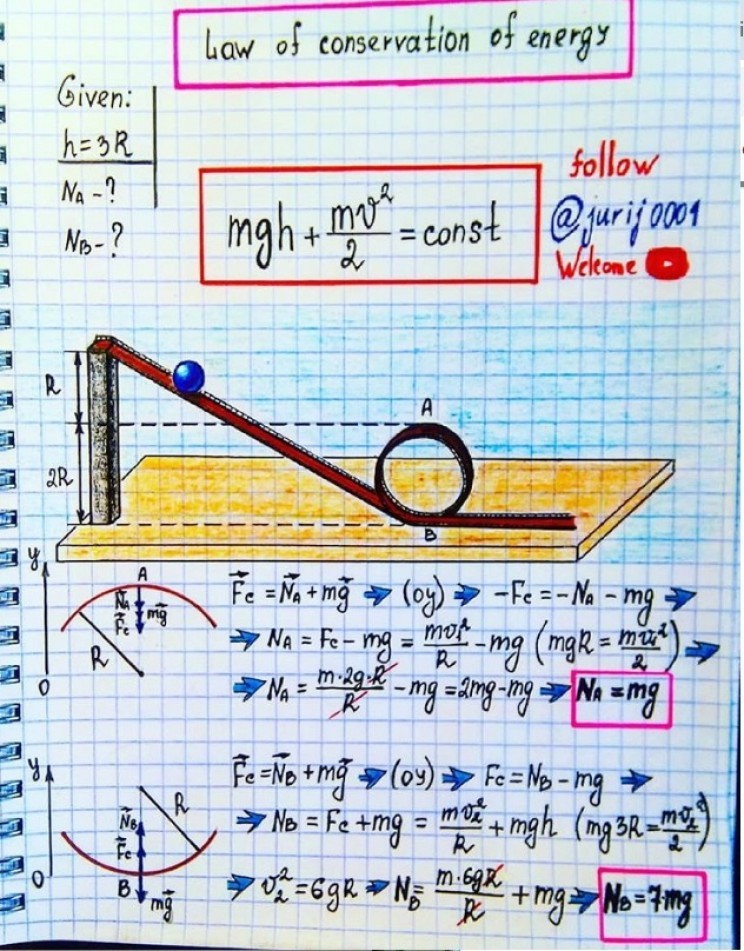### 5. Electromagnetism

What exactly is electromagnetism? Well, this illustration explains it pretty well. “Length of the movable conductor AB is equal to l. Its resistance is R. the resistance of the stationary conductor through which slides the conductor AB, is negligible. Perpendicular to the plane of the conductors applied magnetic field B. What force F must be applied to the conductor AB, so that it moves at a constant speed v. The system of conductors is in the horizontal plane.”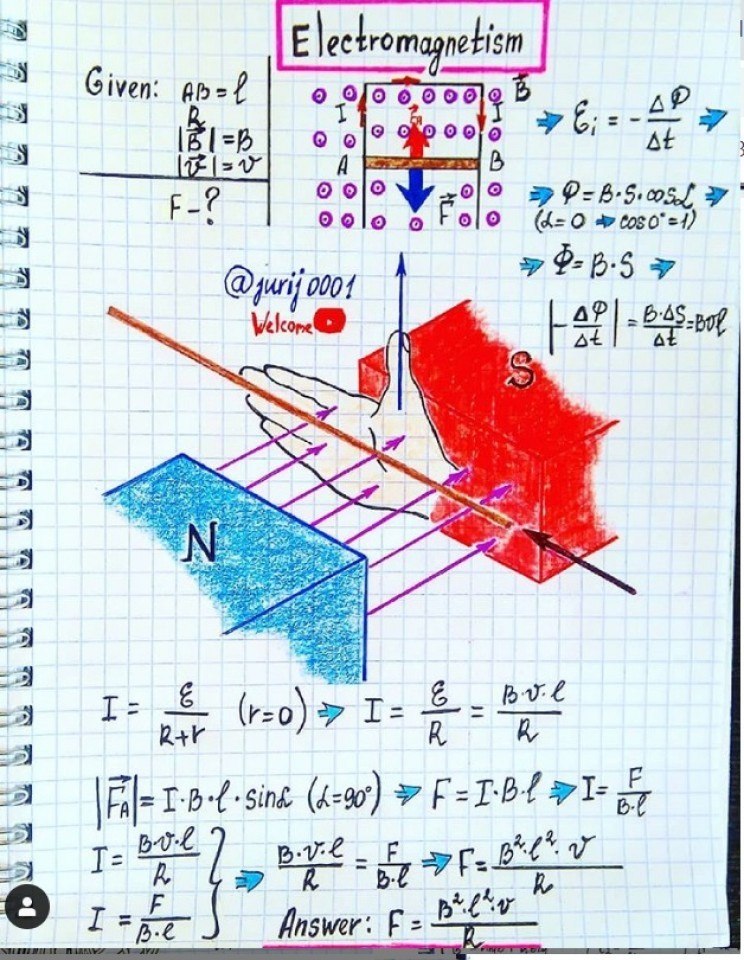## Two U.S. Air Force Fifth-Generation Fighter Jet From Eglin Air Force Base Has Crashed In Last Four Days

Lockheed Martin F-22 Raptor and F-35 Lightning II. © Steve Thorne © Getty Images For the second …